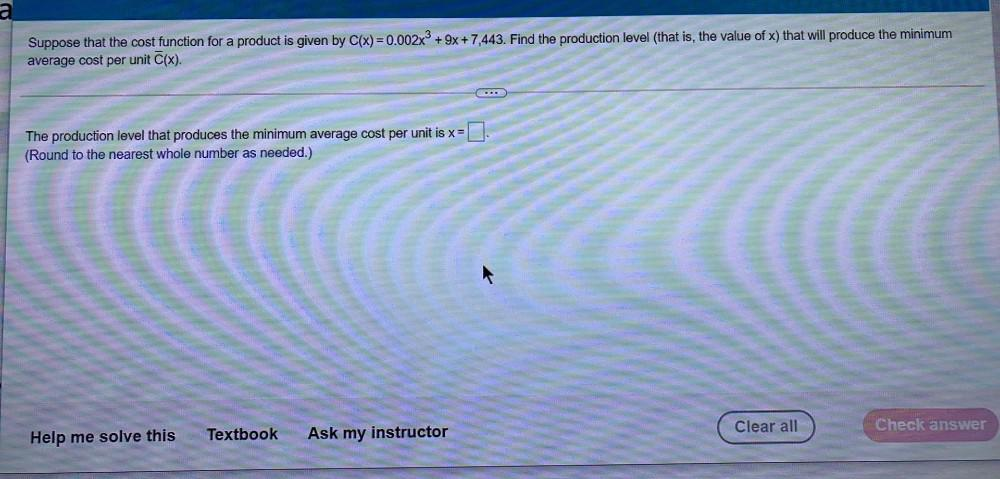Question:

# a Suppose that the cost function for a product is given by C(x)=0.002x3 + 9x + 7,443. Find the production level (that is, the vaa Suppose that the cost function for a product is given by C(x)=0.002x3 + 9x + 7,443. Find the production level (that is, the value of x) that will produce the minimum average cost per unit (x). The production level that produces the minimum average cost per unit is x= (Round to the nearest whole number as needed.) Clear all Check answer Help me solve this Textbook Ask my instructor